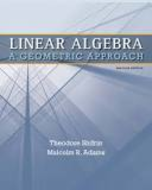×

×

Textbooks / Math / Linear Algebra: A Geometric Approach 2

# Linear Algebra: A Geometric Approach 2nd Edition - Solutions by Chapter## Full solutions for Linear Algebra: A Geometric Approach | 2nd Edition

ISBN: 9781429215213Linear Algebra: A Geometric Approach | 2nd Edition - Solutions by Chapter

Solutions by Chapter
4 5 0 260 Reviews
##### ISBN: 9781429215213

This textbook survival guide was created for the textbook: Linear Algebra: A Geometric Approach, edition: 2. This expansive textbook survival guide covers the following chapters: 31. The full step-by-step solution to problem in Linear Algebra: A Geometric Approach were answered by , our top Math solution expert on 03/15/18, 05:30PM. Linear Algebra: A Geometric Approach was written by and is associated to the ISBN: 9781429215213. Since problems from 31 chapters in Linear Algebra: A Geometric Approach have been answered, more than 34786 students have viewed full step-by-step answer.

Key Math Terms and definitions covered in this textbook
• Associative Law (AB)C = A(BC).

Parentheses can be removed to leave ABC.

• Cayley-Hamilton Theorem.

peA) = det(A - AI) has peA) = zero matrix.

• Change of basis matrix M.

The old basis vectors v j are combinations L mij Wi of the new basis vectors. The coordinates of CI VI + ... + cnvn = dl wI + ... + dn Wn are related by d = M c. (For n = 2 set VI = mll WI +m21 W2, V2 = m12WI +m22w2.)

• Cofactor Cij.

Remove row i and column j; multiply the determinant by (-I)i + j •

• Commuting matrices AB = BA.

If diagonalizable, they share n eigenvectors.

• Covariance matrix:E.

When random variables Xi have mean = average value = 0, their covariances "'£ ij are the averages of XiX j. With means Xi, the matrix :E = mean of (x - x) (x - x) T is positive (semi)definite; :E is diagonal if the Xi are independent.

• Determinant IAI = det(A).

Defined by det I = 1, sign reversal for row exchange, and linearity in each row. Then IAI = 0 when A is singular. Also IABI = IAIIBI and

• Gram-Schmidt orthogonalization A = QR.

Independent columns in A, orthonormal columns in Q. Each column q j of Q is a combination of the first j columns of A (and conversely, so R is upper triangular). Convention: diag(R) > o.

• Hessenberg matrix H.

Triangular matrix with one extra nonzero adjacent diagonal.

• Multiplication Ax

= Xl (column 1) + ... + xn(column n) = combination of columns.

• Normal equation AT Ax = ATb.

Gives the least squares solution to Ax = b if A has full rank n (independent columns). The equation says that (columns of A)·(b - Ax) = o.

• Right inverse A+.

If A has full row rank m, then A+ = AT(AAT)-l has AA+ = 1m.

• Singular Value Decomposition

(SVD) A = U:E VT = (orthogonal) ( diag)( orthogonal) First r columns of U and V are orthonormal bases of C (A) and C (AT), AVi = O'iUi with singular value O'i > O. Last columns are orthonormal bases of nullspaces.

• Skew-symmetric matrix K.

The transpose is -K, since Kij = -Kji. Eigenvalues are pure imaginary, eigenvectors are orthogonal, eKt is an orthogonal matrix.

• Spanning set.

Combinations of VI, ... ,Vm fill the space. The columns of A span C (A)!

• Subspace S of V.

Any vector space inside V, including V and Z = {zero vector only}.

• Sum V + W of subs paces.

Space of all (v in V) + (w in W). Direct sum: V n W = to}.

• Unitary matrix UH = U T = U-I.

Orthonormal columns (complex analog of Q).

• Vandermonde matrix V.

V c = b gives coefficients of p(x) = Co + ... + Cn_IXn- 1 with P(Xi) = bi. Vij = (Xi)j-I and det V = product of (Xk - Xi) for k > i.

• Volume of box.

The rows (or the columns) of A generate a box with volume I det(A) I.